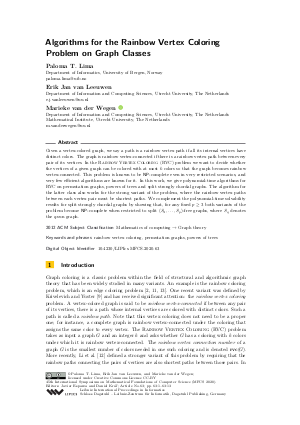Document# Algorithms for the Rainbow Vertex Coloring Problem on Graph Classes

### Authors Paloma T. Lima, Erik Jan van Leeuwen, Marieke van der Wegen## File

LIPIcs.MFCS.2020.63.pdf
• Filesize: 0.51 MB
• 13 pages

## Cite As

Paloma T. Lima, Erik Jan van Leeuwen, and Marieke van der Wegen. Algorithms for the Rainbow Vertex Coloring Problem on Graph Classes. In 45th International Symposium on Mathematical Foundations of Computer Science (MFCS 2020). Leibniz International Proceedings in Informatics (LIPIcs), Volume 170, pp. 63:1-63:13, Schloss Dagstuhl - Leibniz-Zentrum für Informatik (2020)
https://doi.org/10.4230/LIPIcs.MFCS.2020.63

## Abstract

Given a vertex-colored graph, we say a path is a rainbow vertex path if all its internal vertices have distinct colors. The graph is rainbow vertex-connected if there is a rainbow vertex path between every pair of its vertices. In the Rainbow Vertex Coloring (RVC) problem we want to decide whether the vertices of a given graph can be colored with at most k colors so that the graph becomes rainbow vertex-connected. This problem is known to be NP-complete even in very restricted scenarios, and very few efficient algorithms are known for it. In this work, we give polynomial-time algorithms for RVC on permutation graphs, powers of trees and split strongly chordal graphs. The algorithm for the latter class also works for the strong variant of the problem, where the rainbow vertex paths between each vertex pair must be shortest paths. We complement the polynomial-time solvability results for split strongly chordal graphs by showing that, for any fixed p ≥ 3 both variants of the problem become NP-complete when restricted to split (S₃,…,S_p)-free graphs, where S_q denotes the q-sun graph.

## Subject Classification

##### ACM Subject Classification
• Mathematics of computing → Graph theory
##### Keywords
• rainbow vertex coloring
• permutation graphs
• powers of trees

## Metrics

• Access Statistics
• Total Accesses (updated on a weekly basis)
0

## References

1. Andreas Brandstädt, F. Dragan, V. Chepoi, and V. Voloshin. Dually chordal graphs. SIAM Journal on Discrete Mathematics, 11:71-77, 2007.2. Gary Chartrand, Garry L Johns, Kathleen A McKeon, and Ping Zhang. Rainbow connection in graphs. Mathematica Bohemica, 133(1):85-98, 2008.3. Lily Chen, Xueliang Li, and Huishu Lian. Further hardness results on the rainbow vertex-connection number of graphs. Theoretical Computer Science, 481:18-23, 2013.4. Lily Chen, Xueliang Li, and Yongtang Shi. The complexity of determining the rainbow vertex-connection of a graph. Theoretical Computer Science, 412(35):4531-4535, 2011.5. Eduard Eiben, Robert Ganian, and Juho Lauri. On the complexity of rainbow coloring problems. Discrete Applied Mathematics, 246:38-48, 2018.6. Petr A. Golovach, Matthew Johnson, Daniël Paulusma, and Jian Song. A survey on the computational complexity of coloring graphs with forbidden subgraphs. Journal of Graph Theory, 84:331-363, 2017.7. P. Heggernes, D. Issac, J. Lauri, P. T. Lima, and E. J. van Leeuwen. Rainbow vertex coloring bipartite graphs and chordal graphs. In Proceedings of MFCS'18, volume 117 of LIPIcs, pages 83:1-83:13, 2018.8. Daniel Král', Jan Kratochvíl, Zsolt Tuza, and Gerhard J. Woeginger. Complexity of coloring graphs without forbidden induced subgraphs. In WG'01, pages 254-262, 2001.9. Michael Krivelevich and Raphael Yuster. The rainbow connection of a graph is (at most) reciprocal to its minimum degree. Journal of Graph Theory, 63(3):185-191, 2010.10. Juho Lauri. Chasing the Rainbow Connection: Hardness, Algorithms, and Bounds. PhD thesis, Tampere University of Technology, 2016.11. X. Li, Y. Shi, and Y. Sun. Rainbow connections of graphs: A survey. Graphs and Combinatorics, 29:1-38, 2013. URL: https://doi.org/10.1007/s00373-012-1243-2.
12. Xueliang Li, Yaping Mao, and Yongtang Shi. The strong rainbow vertex-connection of graphs. Utilitas Mathematica, 93:213-223, 2014.13. Xueliang Li and Yuefang Sun. An updated survey on rainbow connections of graphs - a dynamic survey. Theory and Applications of Graphs, 0(1):1-38, 2017. URL: https://doi.org/10.20429/tag.2017.000103.
14. Ross M. McConnell and Jeremy P. Spinrad. Modular decomposition and transitive orientation. Discrete Mathematics, 201(1):189-241, 1999. URL: https://doi.org/10.1016/S0012-365X(98)00319-7.
15. Sukumar Mondal, Madhumangal Pal, and Tapan K. Pal. An optimal algorithm to solve the all-pairs shortest paths problem on permutation graphs. Journal of Mathematical Modelling and Algorithms, 2:57-65, 2003. URL: https://doi.org/10.1023/A:1023695531209.
16. L. Sunil Chandran, Anita Das, Deepak Rajendraprasad, and Nithin M. Varma. Rainbow connection number and connected dominating sets. Journal of Graph Theory, 71(2):206-218, 2012. URL: https://doi.org/10.1002/jgt.20643.
X

Feedback for Dagstuhl Publishing